Today: parsing, while loop vs. for loop, parse words out of string patterns, boolean precedence, variables

## Data and Parsing

Here's some fun looking data...

```\$GPGGA,005328.000,3726.1389,N,12210.2515,W,2,07,1.3,22.5,M,-25.7,M,2.0,0000*70
\$GPGSA,M,3,09,23,07,16,30,03,27,,,,,,2.3,1.3,1.9*38
\$GPRMC,005328.000,A,3726.1389,N,12210.2515,W,0.00,256.18,221217,,,D*78
\$GPGGA,005329.000,3726.1389,N,12210.2515,W,2,07,1.3,22.5,M,-25.7,M,2.0,0000*71
\$GPGSA,M,3,09,23,07,16,30,03,27,,,,,,2.3,1.3,1.9*38
\$GPRMC,005329.000,A,3726.1389,N,12210.2515,W,0.00,256.18,221217,,,D*79
\$GPGGA,005330.000,3726.1389,N,12210.2515,W,2,07,1.3,22.5,M,-25.7,M,3.0,0000*78
\$GPGSA,M,3,09,23,07,16,30,03,27,,,,,,2.3,1.3,1.9*38
...
```
• The above is what a GPS chip outputs
-buried deep in your phone, this is going on
• A standard: NMEA
- NMEA_018 (wikipedia)
• Notice: it's just text
-a series of text lines ending with \n
-each line is made of chars
• Text is a super common exchange format between systems
• "Parsing"
-Have "raw" text like this
-Find and pull out the data you want
• On the surface, we are doing parsing examples
• In reality, learning index/loop algorithms, parsing is just the domain

## Foreshadow: Advance With `var += 1`

• Framing for today's example
• Imagine a string on paper
• Finger is pointing at a char
• Move finger to the right, looking for something
• Python: have a var `end` with an index into string
• e.g. `end` is 4, pointing at the `'a'`
• `end += 1` .. like moving one to the right
• Continue `end += 1` until get to a space

Start with `end = 4`. Advance to space char with `end += 1` in loop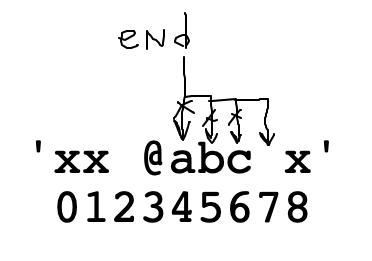## `for i/range` vs. `while`

The for/i/range form is great for going through numbers which you know ahead of time - a common pattern in real programs. If you need to go through `0..n-1` - use for/i/range, that's exactly what it's for.

```for i in range(n):
# i is 0, 1, 2, .. n-1
```

## `while` Loop - Flexible

But we also have the while loop. The "for" is suited for the case where you know the numbers ahead of time. The while is more flexible. The while can test on each iteration, stop at the right spot. Ultimately you need both forms, but here we will switch to using while.

## while Equivalent of for/range

It's possible to write the equivalent of for/i/range as a while loop instead. This is not a good way to go through `0..n-1`, but it does show a way to structure a while loop.

• `for i in range(n)` - go-to solution for that sequence
• Can write this as a `while` .. do steps manually
• Three parts: init, test, update
• Use range() for common `0..n-1` case
• Use while where need fine control of i (examples to follow)
• Beware: easy to forget update step, result is infinite loop
for/range is so common .. we don't have muscle-memory for the update line

Here is the while-equivalent to `for i in range(n)`

```i = 0         # 1. init
while i < n:  # 2. test
# use i
i += 1    # 3. update, loop-bottom
# (easy to forget this line)
```

## Example while_double()

> while_double() (in parse1 section)

double_char() written as a while. The for-loops is the correct approach here, so here just showing for-while equivalence.

```def while_double(s):
result = ''
i = 0
while i < len(s):
result += s[i] + s[i]
i += 1
return result
```

## Index is Valid: `i < length`

With zero based indexing, if we are increasing an index variable `i`, then `i < length` is the easy test that `i` is a valid index; that it is not too big.

Look at our old `'Python'` str exampleIf we are increasing an index number, `5` is the last valid index. When we increase it to `6` it's past the end of the string. The length here is `6`, so in effect `i < 6` checks that `i` is valid if we are increasing `i`.

If we are decreasing `i`, then `i >= 0` is the valid check, since `0` is the first index.

## Example: at_word()

> at_word() (in parse1 section)

```'xx @abcd xyz' -> 'abcd'
'x@ab^xyz' -> 'ab'
```

at_word(s): We'll say an at-word is an '@' followed by zero or more alphabetic chars. Find and return the alphabetic part of the first at-word in s, or the empty string if there is none. So 'xx @abc xyz' returns 'abc'.

• Realistic parsing problem, extracting the wanted part of a string
• Demonstrate several patterns on this one
• We'll re-use these patterns
• We'll work through this one carefully
Use str.find() to locate each @
Use while to skip over alpha chars to find end
Use `var < len(s)` to protect use of `s[var]`
Var names: search, at, end - try to keep things straight

## at_word() Strategy 1

First use `s.find()` to locate the `'@'`. Then start end pointing to the right of the `'@'`.

Start of loop: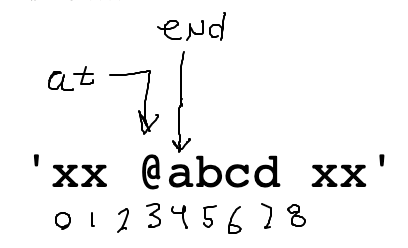```    at = s.find('@')
if at == -1:
return ''

end = at + 1
```

## at_word() Strategy 2 - Loop

Use a while loop to advance end over the alphabetic chars. Make a drawing below to sketch out this strategy.

• AKA skip over the alpha chars
• Loop test: this is true = advance end by one
• Test: `s[end].isalpha()`
• Reminisce : Bit "true = go" pattern for moving forward
• This code will 90% work, with one case to fix later
• Loop leaves end pointing to the first non-alpha char

End of loop: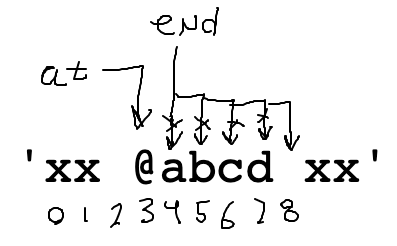```    # Advance end over alpha chars
while s[end].isalpha():
end += 1
```

## at_word() Slice with end

Once we have at/end computed, pulling out the result word is just a slice.

```    word = s[at + 1:end]
return word
```

## at_word() V1

Put those phrases together and it's an excellent first try, and it 90% works. Run it.

```def at_word(s):
at = s.find('@')
if at == -1:
return ''

end = at + 1
# Advance end over alpha chars
while s[end].isalpha():
end += 1

word = s[at + 1:end]
return word
```

## at_word: 'woot' Bug

That code is pretty good, but there is actually a bug in the while-loop. It has to do with particular form of input case below, where the alphabetic chars go right up to the end of the string. Think about how the loop works when advancing "end" for the case below.

```    at = s.find('@')
end = at + 1
while s[end].isalpha():
end += 1
```
```

'xx@woot'
01234567
```

Problem: keep advancing "end" .. past the end of the string, eventually end is 7. Then the while-test `s[end].isalpha()` throws an error since index 7 is past the end of the string.

The loop above translates to: "advance end so long as `s[end]` is alphabetic"

To fix the bug, we modify the test to: "advance end so long as `end` is valid and `s[end]` alphabetic".

In other words, stop advancing if end reaches the end of the string.

Loop end bug: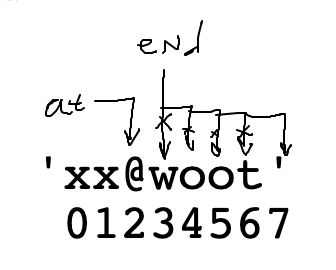## Solution: `end < len(s)` Guard Test

This "guard" pattern will be a standard part of looping over something. We cannot access `s[end]` when end is too big. Add a "guard" test `end < len(s)` before the `s[end]`. This stops the loop when end gets to 7. The slice then works as before. This code is correct.

```def at_word(s):
at = s.find('@')
if at == -1:
return ''

# Advance end over alpha chars
end = at + 1
while end < len(s) and s[end].isalpha():
end += 1

word = s[at + 1:end]
return word
```

## Guard / Short Circuit Pattern

The "and" evaluates left to right. As soon as it sees a `False` it stops. In this way the `< len(s)` guard checks that "end" is a valid number, before `s[end]` tries to use it. This a standard pattern: the index-is-valid guard is first, then "and", then `s[end]` that uses the index. We'll see more examples of this guard pattern.

## Fix End Bug Recap

• Bug: run end off the end of s, testing non-existent `s[end]`
• e.g. this happens if input is `s = 'xx @woot'`
Think through how the loop works for that case
• Solution:
• Add guard `<`
• This is the fixed loop:
`while end < len(s) and s[end].isalpha():`
• Q: How to test if index `i` is valid in `s`?
• A: `i < len(s)`
• Only look at `s[end]` char after checking that `end` is valid
• Boolean Short Circuit
Python evaluates expression left-right
As soon as boolean value determined, stops trying
A `False` in the midst of an `and` stops
So the `<` guards the `s[end].isalpha()`
• Common guard pattern:
Check `i < len(s)` before trying `s[i]`

## `s[end]` vs. `s[at + 1:end]`

• Accessing `s[end]` off the end of the string is an error
So we need the guard
• What about the slice `s[at + 1:end]`
• That actually works fine, for two reasons...

## Reason 1 - UBNI

• Why does `s[at + 1:end]` work fine?
• Up To But Not Including - UBNI
• The char at the second index is not included in the slice
• So the fact that it's one past the end is fine - it is not included
• This is the best reason, so focus on this one

## Reason 2 - Slice Garbage

• The other reason it works is a little sketchy
• It turns out, slices never raise an error about bad out of bounds index numbers
• They will work with any old garbage numbers
• If a number is too big, it is interpreted as "the end of the string"
• This does not mean you can stop caring about index numbers
• It just means the slice is not checking for you
• Our above solution is fine - the `end` index is managed accurately
Going exactly one past the chars we want in all cases
```>>> s = 'Python'
>>> len(s)
6
>>> s[2:5]
'tho'
>>> s[2:6]
'thon'
>>> s[2:46789]
'thon'
```

## at_words() - Zero Char Case - Works?

• What about `'xx @ xx'`
• Consider slice of @ above
`s[at + 1:end]`
Turns out to be like `s[4:4]`
Which is the empty string `''`, so the code we have works perfectly for this edge case

## Example exclamation()

exclamation(s): We'll say an exclamation is zero or more alphabetic chars ending with a '!'. Find and return the first exclamation in s, or the empty string if there is none. So `'xx hi! xx'` returns `'hi!'`. (Like at_word, but right-to-left).

Will need a guard here, as the loop goes right-to-left. The leftmost valid index is 0, so that will figure in the guard test.

## exclamation() Solution

```def exclamation(s):
exclaim = s.find('!')
if exclaim == -1:
return ''

# Move start left over alpha chars
# guard: start >= 0
start = exclaim - 1
while start >= 0 and s[start].isalpha():
start -= 1

# start is on the first *non* alpha
word = s[start + 1:exclaim + 1]
return word
```

## Recall: Boolean Operators

• Arithmetic operators: + - * /
• Combine numbers to make a number
`1 + 2 * 3 -> 7`
• Boolean operators: `and or not`
• Combine Boolean values to make a Boolean
`True or False -> True`
`True and False -> False`
`True and Not False -> True`

## Boolean Expressions

See the guide for details Boolean Expression

• Boolean operators: `and or not`
• Mixture of these, can add parenthesis to force order of operation
• Say have these variables:
`age` - int age, say age is good if less than 30
`is_raining` - boolean, True if raining
`is_weekend`- boolean, True if it's the weekend
• Definition of a good day, need two things:
1. it must not be raining. This is mandatory.
2. then either age is under 30 or it's the weekend

The code below looks reasonable, but doesn't quite work right

```def good_day(age, is_weekend, is_raining):
if not is_raining and age < 30 or is_weekend:
print('good day')
```

## Boolean Precedence:

• `not` = highest, (like - in -7)
• `and` = next highest (like *)
• `or` = lowest (like +)

## What The Above Does

Because and is higher precedence than or as written above, the code above acts like the following (the and going before the or):

```   if (not is_raining and age < 30) or is_weekend:
```

What is a set of data that this code will evaluate incorrectly? raining=True, age=anything, weekend=True .. the `or weekend` makes the whole thing True, no matter what the other values are. This does not match the good-day definition above, which requires that it not be raining.

## Boolean Precedence Solution

The solution we will spell out is not difficult.

• Many programmers do not have boolean precedence memorized .. fine
• Do remember that "not" is the highest precedence
• Solution: note when you have a mixture of and + or
When there is a mixture, the precedence will matter
put in parenthesis in that case
• We will never complain about extra parenthesis, so just add them to spell out the order you want
• In this case, put parens to group the or part, separating from not-raining
• BTW similar logic applies to math - if there's a mixture of * and +, add parenthesis

Solution

```def good_day(age, is_weekend, is_raining):
if not is_raining and (age < 30 or is_weekend):
print('good day')
```

## Parse "or" Example - at_word99()

This is operating at a realistic level for parsing data.

at_word99(): Like at-word, but with digits added. We'll say an at-word is an '@' followed by zero or more alphabetic or digit chars. Find and return the alpha-digit part of the first at-word in s, or the empty string if there is none. So 'xx @ab12 xyz' returns 'ab12'.

## "end" Loop For at_words99()

Like before, but now a word is made of alpha or digit - many real problems will need this sort of code. This may be our most complicated line of code thus far in the quarter! Fortunately, it's a re-usable pattern for any of these "find end of xxx chars" problems.

The most difficult part is the "end" loop to locate where the word ends. What is the while test here? (Bring up at_word99() in other window to work it out). We want to use "or" to allow alpha or digit.

```at = s.find('@')
end = at + 1
while ??????????:
end += 1
```## at_word99() While Test

```# 1. Still have the < guard
# 2. Use "or" to allow isalpha() or isdigit()
# 3. Need to add parens, since this has and+or
#    combination
while end < len(s) and (s[end].isalpha() or s[end].isdigit()):
end += 1
```

## at_word99() Solution

```def at_word99(s):
at = s.find('@')
if at == -1:
return ''

# Advance end over alpha or digit chars
# use "or" + parens
end = at + 1
while end < len(s) and (s[end].isalpha() or s[end].isdigit()):
end += 1

word = s[at + 1:end]
return word
```

If we have time, we'll do this bit.

## We Need To Have a Little Talk About Variable Names

With the following code, it's clear that the assignment `=` sets the variable to point to a value.

```x = 7
```

## For Loop Sets Variables Too

It's less obvious, but the for loop just sets a variable too, once for each iteration. The variable name is the word the programmer chooses right after the word "for", in this example the variable is `i` which is an idiomatic choice:

```for i in range(4):
# use i
print(i)

0
1
2
3
```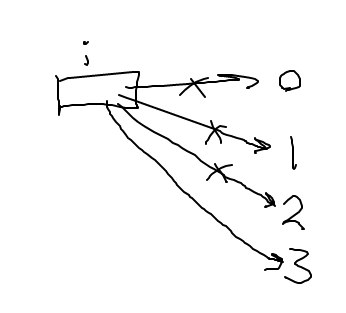## Variables and Meaninglessness

The Sartre of Coding!

The variable name is just the label applied to the box that hold the pointer.

You might get the feeling in CS106A to this point: it will only work if the variable is named "i", but that's not true. We always name it "i" since that's the idiom programmers use for that context, so you cannot be blamed for thinking it was some Python rule.

We try to choose a sensible label to keep our own thoughts organized. However the computer does not care about the word used, so long as the word chosen is used consistently across lines. The variable name `i` is idiomatic for that sort of loop. But in reality we could use any variable name, and the code would work exactly the same. Say we name the variable `meh` instead .. same output. All that matters is that the variable on line 1 is the same as on line 2.

```for meh in range(4):
print(meh)

0
1
2
3
```This is a little disturbing. We do try to choose good and/or idiomatic variable names for our own sake. However, the computer does not notice or care about the actual word choice for our variables. The computer does not understand English here; it just recognizes that two words are the same and so must be the same variable.